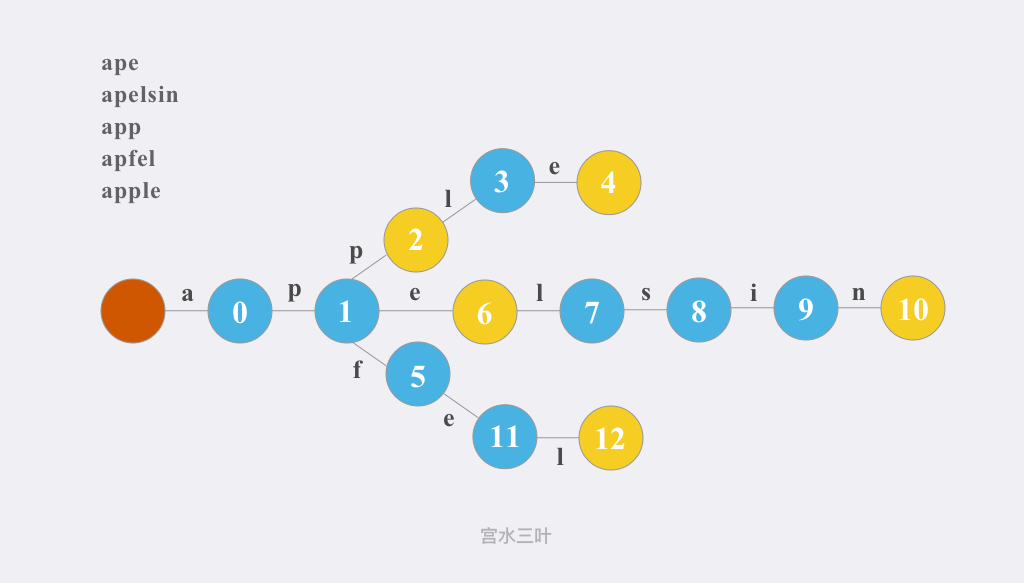# LC 211. 添加与搜索单词 - 数据结构设计

### 题目描述

• WordDictionary() 初始化词典对象
• void addWord(word)word 添加到数据结构中，之后可以对它进行匹配
• bool search(word) 如果数据结构中存在字符串与 word 匹配，则返回 true ；否则，返回 false
• word 中可能包含一些 '.' ，每个 . 都可以表示任何一个字母。

• $1 <= word.length <= 500$
• addWord 中的 word 由小写英文字母组成
• search 中的 word'.' 或小写英文字母组成
• 最多调用 $50000$ 次 addWordsearch

### 基本分析

$Trie$ 树（又叫「前缀树」或「字典树」）是一种用于快速查询「某个字符串/字符前缀」是否存在的数据结构。### 二维数组

• 时间复杂度：$L$ 为字符串的最大长度，addWord 操作的复杂度为 $O(L)$；search 操作的复杂度为 $O(C * L)$，其中 $C$ 为字符集大小，固定为 $26$
• 空间复杂度：静态数组大小固定，复杂度为 $O(1e7)$

### TrieNode

• 时间复杂度：addWord 操作的复杂度为 $O(L)$；search 操作的复杂度为 $O(C * L)$，其中 $C$ 为字符集大小，固定为 $26$
• 空间复杂度：令 n 为插入字符串数量，$L$ 为字符串的最大长度，复杂度为 $O(n * L)$